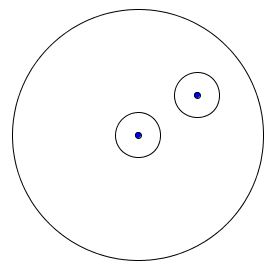# Cutting Circle to Rearrange Two Dots

### Problem

In addition to the center of a circle another dot is marked within. Cut the circle into at most three parts such that, by rearranging the pieces, you get a circle with the center at the dot.### Solution 1

A three pieces solution: Cut off equal circles - small enough to fit within the circle - around the center and the given dot. Exchange the two small circles.### Solution 2

A two pieces solution: Draw equal circles - small enough to fit within the circle - around the center and the given dot. Draw the common external tangents to the two circle. Cut off so obtained shape, turn it around and fit it back in.### Solution 3

Around the given dot draw a circle (an arc actually) with the radius of the given circle. Cut along the arc. The given circle will be split into a lens-like and a crescent-like shapes. Slider the latter around the lens.### References

1. S. Dorichenko, A Moscow Math Circle: Week-by-week Problem Sets, MSRI/AMS, 2012, #0.4

### Generalization

What if both of the given points are marked randomly in the circle? How many cuts does it take to exchange the two points?The first two solutions will clearly work in this case as well. What about the third solution? Can the third solution be modified to solve the more general problem?